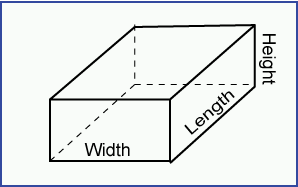created: 09/23/99; revised 07/04/2011, 08/18/2017

# Fill in the Blanks1. Design of the Box Class. Objects of this class will represent a box (such as a cardboard box.) The sides of a box are rectangles. A Box will have three essential characteristics: width, height, and length. From these characteristics other values may be calculated: volume and total surface area.

Fill in the blanks in the following design for the class:

public class

A class that models a cardboard box.

Constructors

```public Box ( double ,
double ,
double   )
```

The parameters can be in any order, as long as you use that order consistently in the rest of the code.

The constructor should not include volume and area as parameters because these values can be calculated from the width, height, and length of the box. As a convenience, include a constructor to use when all sides of the box are the same size (when it is a cube):

```public Box ( double  )
```

Methods

```// calculate the volume of the box
public double

// calculate the total surface area of the box
public double
```

2. Checking the Design. To check the design, write a small program that uses the class. Write a program that creates a Box object with sides of 2.5, 5.0, and 6.0 inches. Write out its surface area and volume.

```
public class BoxTester
{

public static void main ( String[] args )
{
Box box = new   ;

System.out.println( "Area: "    + box. +
"  volume: " + box. );

}
}
```

3. Skeleton of the Class. Fill in the blanks that give the over-all design of the class.

```public class Box
{
//

//

//

}
```

4. Fill in Instance Variables. Fill in the data type of each instance variable.

```public class Box
{
// Instance Variables
private  length ;
private  width ;
private  height ;

// Constructors

// Methods

}
```

The variables should be floating point because integer dimensions would be too much of a restriction. Nearly always, if you use floating point you should use `double`.

5. Complete the Constructors. Constructors initialize the instance variables of the objects being constructed.

```public class Box
{
// Instance Variables
private double length ;
private double width  ;
private double height ;

// Constructors
public ( double , double , double   )
{
this.width   =   ;
this.height  =  ;
this.length  =  ;
}

// initialize all sides to the same value
public ( double  )
{
width   =  ;
height  =  ;
length  =  ;
}

// Methods

}
```

When an instance variable and a parameter use the same identifier, you specify the instance variable of the object by saying `this.identifier` as in the above.

6. Complete a Method. The specification for the `volume( )` method says it looks like this:

```// calculate the volume of the box
public double  volume()
```

The formula to use is: volume = product of all three sides

```public class Box
{
// Instance Variables
private double length ;
private double width  ;
private double height ;

// Constructors
public Box ( double width, double height, double length )
{
this.width  = width ;
this.height = height ;
this.length = length ;
}

public Box ( double side )
{
width  = side ;
height = side ;
length = side ;
}

// Methods
public double volume()
{
return  *  * ;
}

}
```

(Of course, the three instance variables could be used in any order in the arithmetic expression.)

7. Complete the other Method. The specification for the `area()` method says it looks like this: ``` double area() ```. There are three pairs of sides to a box. Opposite sides have the same area, so the calculation looks like this: ``` 2 * (sum of area of each unique side) ```

```public class Box
{
// Instance Variables
private double length ;
private double width  ;
private double height ;

// Constructors
public Box ( double width, double height, double length )
{
this.width  = width ;
this.height = height ;
this.length = length ;
}

public Box ( double side )
{
width  = side ;
height = side ;
length = side ;
}

// Methods
public double volume()
{
return width * height * length ;
}

public double area()
{
return 2 * (  *  +
*  +
*  ) ;
}

}
```

8. Testing Class. At this point all the coding for the definition of `Box` is done. Say that the small test program (class `BoxTester`) is in a file called `BoxTester.java` and that the complete definition of the `Box` class is in a file called `Box.java`.

```public class BoxTester
{

public static void main ( String[] args )
{

// create a box with sides= 2.5, 3.0, and 5.0

Box box1 = new  Box( 2.5, 3.0, 5.0 );

System.out.println( "Box1 Area: "    + box1.area() +
"  Volume: " + box1.volume()  );

// create a box with all sides = 3.0

Box box2 =   Box (  );

System.out.println( "Box2 Area: "    + box2.area() +
"  Volume: " + box2.volume()  );

}
}
```

9. Compile each File. Each of the two files must be compiled:

```C:\MyFiles>javac

C:\MyFiles>javac
```

10. Run the Program. The java bytecode interpreter must be started with the *.class file that contains the main() method:

```C:\MyFiles>java
```

End of the Exercise. If you want to do it again, click on "Refresh" in your browser window.